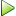Documentation

### This is machine translation

Mouseover text to see original. Click the button below to return to the English version of the page.

## Programming and Scripts

The simplest type of MATLAB® program is called a script. A script is a file that contains multiple sequential lines of MATLAB commands and function calls. You can run a script by typing its name at the command line.

### Scripts

To create a script, use the `edit` command,

`edit mysphere`

This command opens a blank file named `mysphere.m`. Enter some code that creates a unit sphere, doubles the radius, and plots the results:

```[x,y,z] = sphere; r = 2; surf(x*r,y*r,z*r) axis equal ```

Next, add code that calculates the surface area and volume of a sphere:

```A = 4*pi*r^2; V = (4/3)*pi*r^3; ```

Whenever you write code, it is a good practice to add comments that describe the code. Comments enable others to understand your code and can refresh your memory when you return to it later. Add comments using the percent (`%`) symbol.

```% Create and plot a sphere with radius r. [x,y,z] = sphere; % Create a unit sphere. r = 2; surf(x*r,y*r,z*r) % Adjust each dimension and plot. axis equal % Use the same scale for each axis. % Find the surface area and volume. A = 4*pi*r^2; V = (4/3)*pi*r^3; ```

Save the file in the current folder. To run the script, type its name at the command line:

`mysphere`

You can also run scripts from the Editor using the button,.

### Live Scripts

Instead of writing code and comments in plain text, you can use formatting options in live scripts to enhance your code. Live scripts allow you to view and interact with both code and output and can include formatted text, equations, and images.

For example, convert `mysphere` to a live script by selecting and changing the file type to a MATLAB live code file (`*.mlx`). Then, replace the code comments with formatted text. For instance:

• Convert the comment lines to text. Select each line that begins with a percent symbol, and then select ,. Remove the percent symbols.

• Rewrite the text to replace the comments at the end of code lines. To apply a monospace font to function names in the text, select. To add an equation, select on the tab.To create a new live script using the `edit` command, include the `.mlx` extension with the file name:

`edit newfile.mlx`

### Loops and Conditional Statements

Within any script, you can define sections of code that either repeat in a loop or conditionally execute. Loops use a `for` or `while` keyword, and conditional statements use `if` or `switch`.

Loops are useful for creating sequences. For example, create a script named `fibseq` that uses a `for` loop to calculate the first 100 numbers of the Fibonacci sequence. In this sequence, the first two numbers are 1, and each subsequent number is the sum of the previous two, Fn = Fn-1 + Fn-2.

```N = 100; f(1) = 1; f(2) = 1; for n = 3:N f(n) = f(n-1) + f(n-2); end f(1:10) ```

When you run the script, the `for` statement defines a counter named `n` that starts at 3. Then, the loop repeatedly assigns to `f(n)`, incrementing `n` on each execution until it reaches 100. The last command in the script, `f(1:10)`, displays the first 10 elements of `f`.

```ans = 1 1 2 3 5 8 13 21 34 55 ```

Conditional statements execute only when given expressions are true. For example, assign a value to a variable depending on the size of a random number: `'low'`, `'medium'`, or `'high'`. In this case, the random number is an integer between 1 and 100.

```num = randi(100) if num < 34 sz = 'low' elseif num < 67 sz = 'medium' else sz = 'high' end ```

The statement `sz = 'high'` only executes when `num` is greater than or equal to 67.

### Script Locations

MATLAB looks for scripts and other files in certain places. To run a script, the file must be in the current folder or in a folder on the search path.

By default, the `MATLAB` folder that the MATLAB Installer creates is on the search path. If you want to store and run programs in another folder, add it to the search path. Select the folder in the Current Folder browser, right-click, and then select Add to Path.